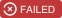Showing:

0

958

2mos ago

18

0

MIT

# SwiftyRSA

Maintainer(s): @ikeithPublic key RSA encryption in Swift.

SwiftyRSA is used in the Scoop iOS app to encrypt driver license numbers before submitting them to Checkr through our API.

## Installation

### Swift 5.0+

SwiftyRSA uses Swift 5.0 and requires Xcode 10.2+.

With Cocoapods:

``````pod 'SwiftyRSA'
``````

With Carthage:

``````github "TakeScoop/SwiftyRSA"
``````

### Objective-C

``````pod 'SwiftyRSA/ObjC'
``````

## Quick Start

### Encrypt with a public key

``````let publicKey = try PublicKey(pemNamed: "public")
let clear = try ClearMessage(string: "Clear Text", using: .utf8)
let encrypted = try clear.encrypted(with: publicKey, padding: .PKCS1)

// Then you can use:
let data = encrypted.data
let base64String = encrypted.base64String
``````

### Decrypt with a private key

``````let privateKey = try PrivateKey(pemNamed: "private")
let encrypted = try EncryptedMessage(base64Encoded: "AAA===")
let clear = try encrypted.decrypted(with: privateKey, padding: .PKCS1)

// Then you can use:
let data = clear.data
let base64String = clear.base64String
let string = clear.string(using: .utf8)
``````

### Create a public/private key representation

#### With a DER file

``````let publicKey = try PublicKey(derNamed: "public")
let privateKey = try PrivateKey(derNamed: "private")
``````

#### With a PEM file

``````let publicKey = try PublicKey(pemNamed: "public")
let privateKey = try PrivateKey(pemNamed: "private")
``````

#### With a PEM string

``````let publicKey = try PublicKey(pemEncoded: str)
let privateKey = try PrivateKey(pemEncoded: str)
``````

#### With a Base64 string

``````let publicKey = try PublicKey(base64Encoded: base64String)
let privateKey = try PrivateKey(base64Encoded: base64String)
``````

#### With data

``````let publicKey = try PublicKey(data: data)
let privateKey = try PrivateKey(data: data)
``````

#### With a SecKey

``````let publicKey = try PublicKey(reference: secKey)
let privateKey = try PrivateKey(reference: secKey)
``````

### Encrypt with a public key

``````let str = "Clear Text"
let clear = try ClearMessage(string: str, using: .utf8)
let encrypted = try clear.encrypted(with: publicKey, padding: .PKCS1)

let data = encrypted.data
let base64String = encrypted.base64Encoded
``````

### Decrypt with a private key

``````let encrypted = try EncryptedMessage(base64Encoded: base64String)
let clear = try encrypted.decrypted(with: privateKey, padding: .PKCS1)

let data = clear.data
let base64String = clear.base64Encoded
let string = try clear.string(using: .utf8)
``````

### Sign with a private key

SwiftyRSA can sign data with a private key. SwiftyRSA will calculate a SHA digest of the supplied `String`/`Data` and use this to generate the digital signature.

``````let clear = try ClearMessage(string: "Clear Text", using: .utf8)
let signature = clear.signed(with: privateKey, digestType: .sha1)

let data = signature.data
let base64String = signature.base64String
``````

### Verify with a public key

SwiftyRSA can verify digital signatures with a public key. SwiftyRSA will calculate a digest of the supplied `String`/`Data` and use this to verify the digital signature.

``````let signature = try Signature(base64Encoded: "AAA===")
let isSuccessful = try clear.verify(with: publicKey, signature: signature, digestType: .sha1)
``````

### Create a public/private RSA key pair

``````let keyPair = SwiftyRSA.generateRSAKeyPair(sizeInBits: 2048)
let privateKey = keyPair.privateKey
let publicKey = keyPair.publicKey
``````

### Export a key or access its content

``````let pem = try key.pemString()
let base64 = try key.base64String()
let data = try key.data()
let reference = key.reference
let originalData = key.originalData
``````

### Use X.509 certificate

SwiftyRSA supports X.509 certificate for public keys. SwiftyRSA can add the X.509 header to a headerless public key, or on the contrary strip it to get a key without a header.

``````let publicKey = PublicKey(data: data)
let publicKeyData = try publicKey.data()
``````

#### Strip the X.509 header from a public key

``````let publicKey_headerLess: Data = try SwiftyRSA.stripKeyHeader(keyData: publicKey_with_X509_header)
``````

Warning : Storing (with SwiftyRSA's methods) or creating a `PublicKey` instance will automatically strip the header from the key. For more info, see Under the hood above.

## Create public and private RSA keys

Use `ssh-keygen` to generate a PEM public key and a PEM private key. SwiftyRSA also supports DER public keys.

``````\$ ssh-keygen -t rsa -f ~/mykey -N ''
\$ cat ~/mykey > ~/private.pem
\$ ssh-keygen -f ~/mykey.pub -e -m pem > ~/public.pem
``````

Your keys are now in `~/public.pem` and `~/private.pem`. Don't forget to move `~/mykey` and `~/mykey.pub` to a secure place.

## Under the hood

To enable using public/private RSA keys on iOS, SwiftyRSA uses a couple techniques like X.509 header stripping so that the keychain accepts them.

When encrypting using a public key:

• If the key is in PEM format, get rid of its meta data and convert it to Data
• Strip the public key X.509 header, otherwise the keychain won't accept it
• Add the public key to the keychain, with a random tag
• Get a reference on the key using the key tag
• Use `SecKeyEncrypt` to encrypt a `ClearMessage` using the key reference and the message data.
• Store the resulting encrypted data to an `EncryptedMessage`
• When the key gets deallocated, delete the public key from the keychain using its tag

When decrypting using a private key:

• Get rid of PEM meta data and convert to Data
• Add the private key to the app keychain, with a random tag
• Get a reference on the key using the key tag
• Use `SecKeyDecrypt` to decrypt an `EncryptedMessage` using the key reference and the encrypted message data
• Store the resulting decrypted data to a `ClearMessage`
• Delete private key from keychain using tag

## Rate & Review

Great Documentation0
Easy to Use0
Performant0
Highly Customizable0
Bleeding Edge0
Responsive Maintainers0
Poor Documentation0
Hard to Use0
Slow0
Buggy0
Abandoned0
Unwelcoming Community0
100